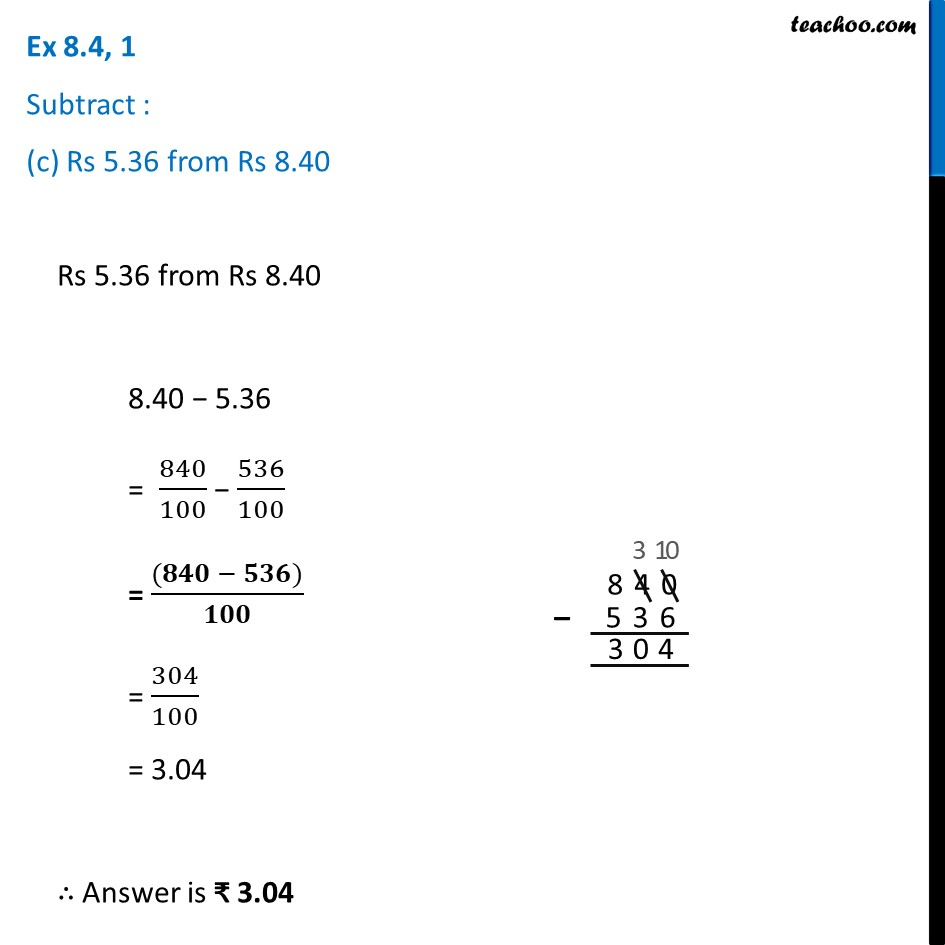Ex 8.4

Chapter 8 Class 6 Decimals
Serial order wiseLearn in your speed, with individual attention - Teachoo Maths 1-on-1 Class

### Transcript

Ex 8.4, 1 Subtract : (c) Rs 5.36 from Rs 8.40 Rs 5.36 from Rs 8.40 8.40 − 5.36 = 840/100 − 536/100 = ((𝟖𝟒𝟎 − 𝟓𝟑𝟔))/𝟏𝟎𝟎 = 304/100 = 3.04 ∴ Answer is ₹ 3.04